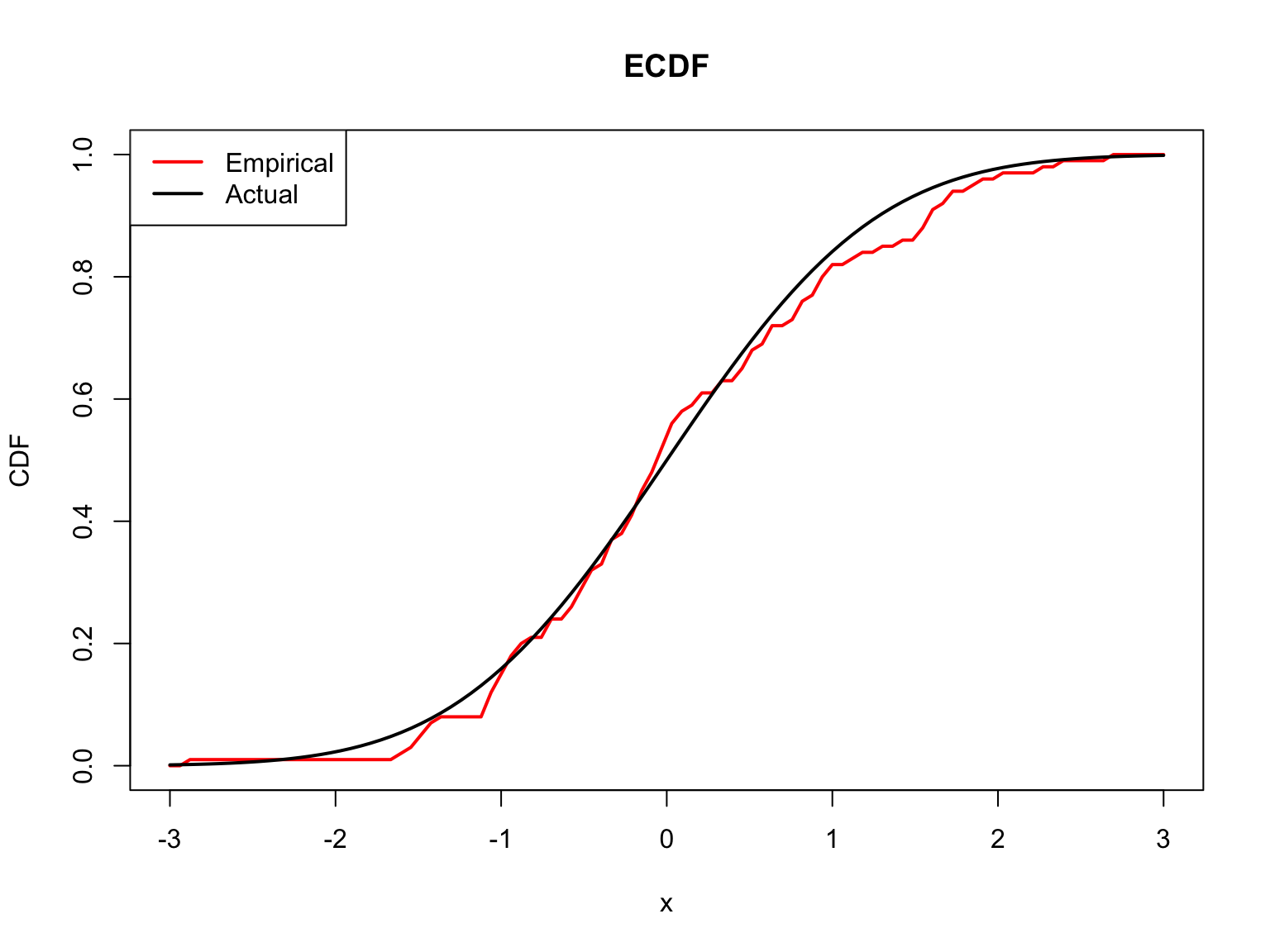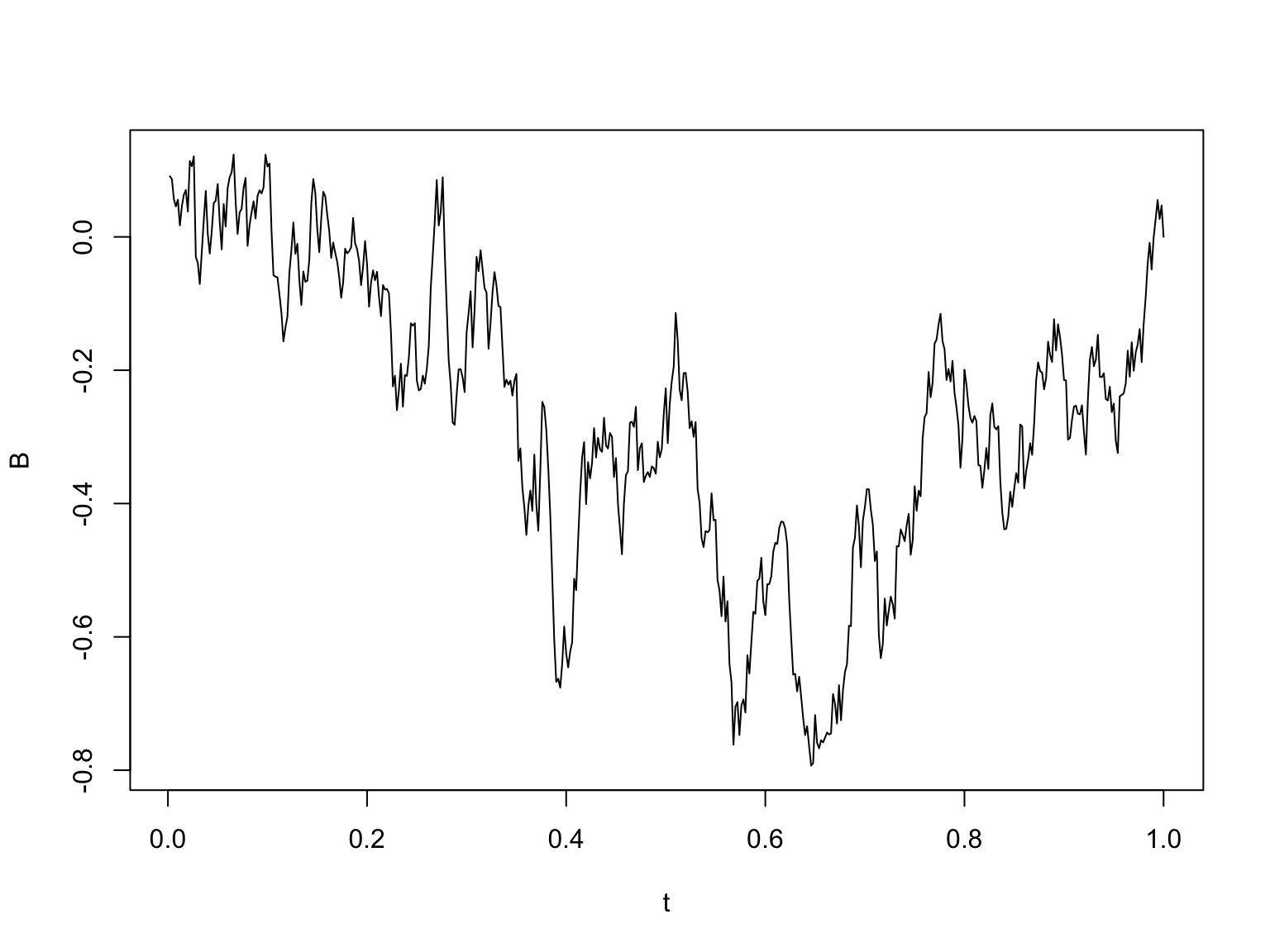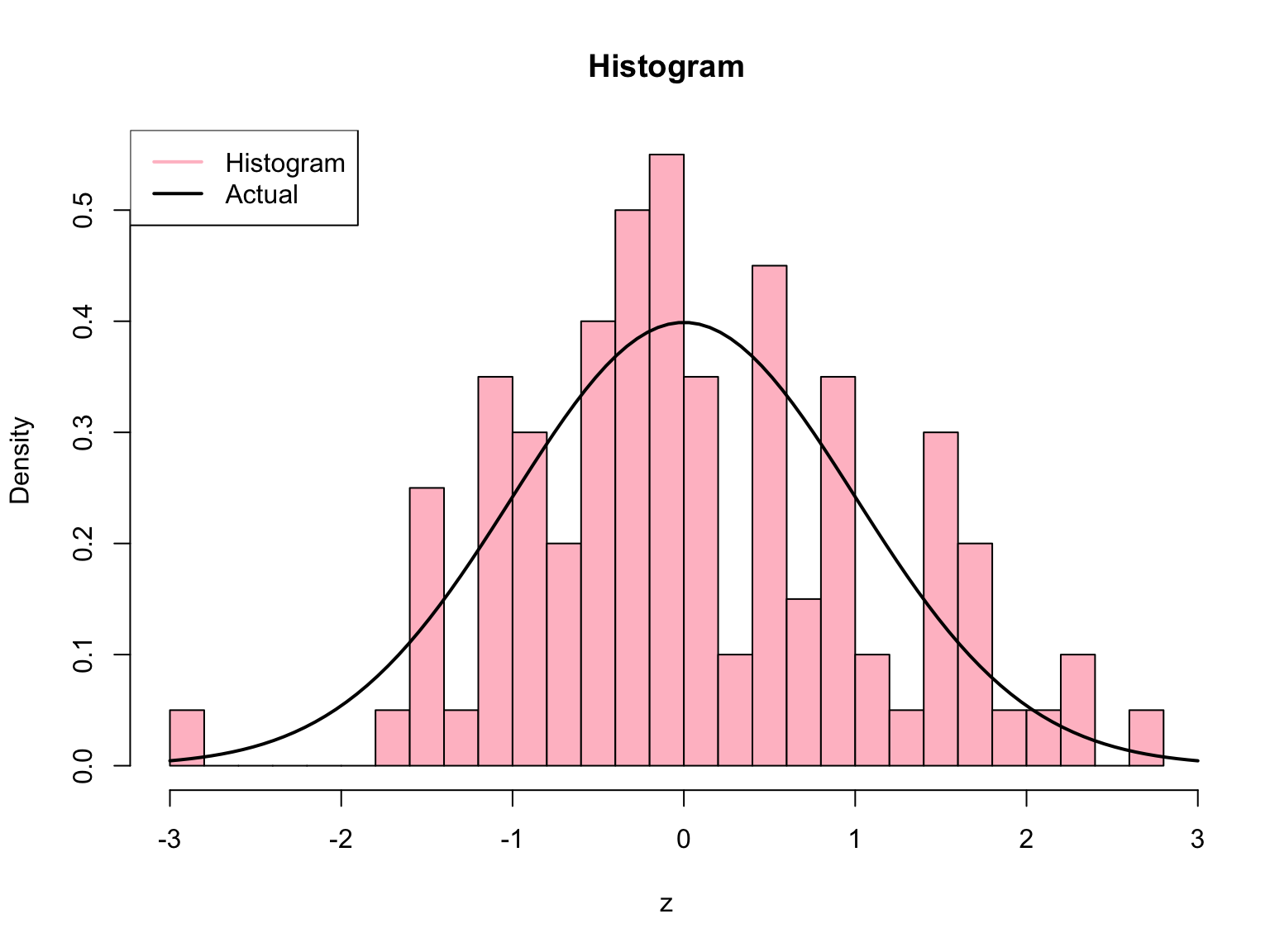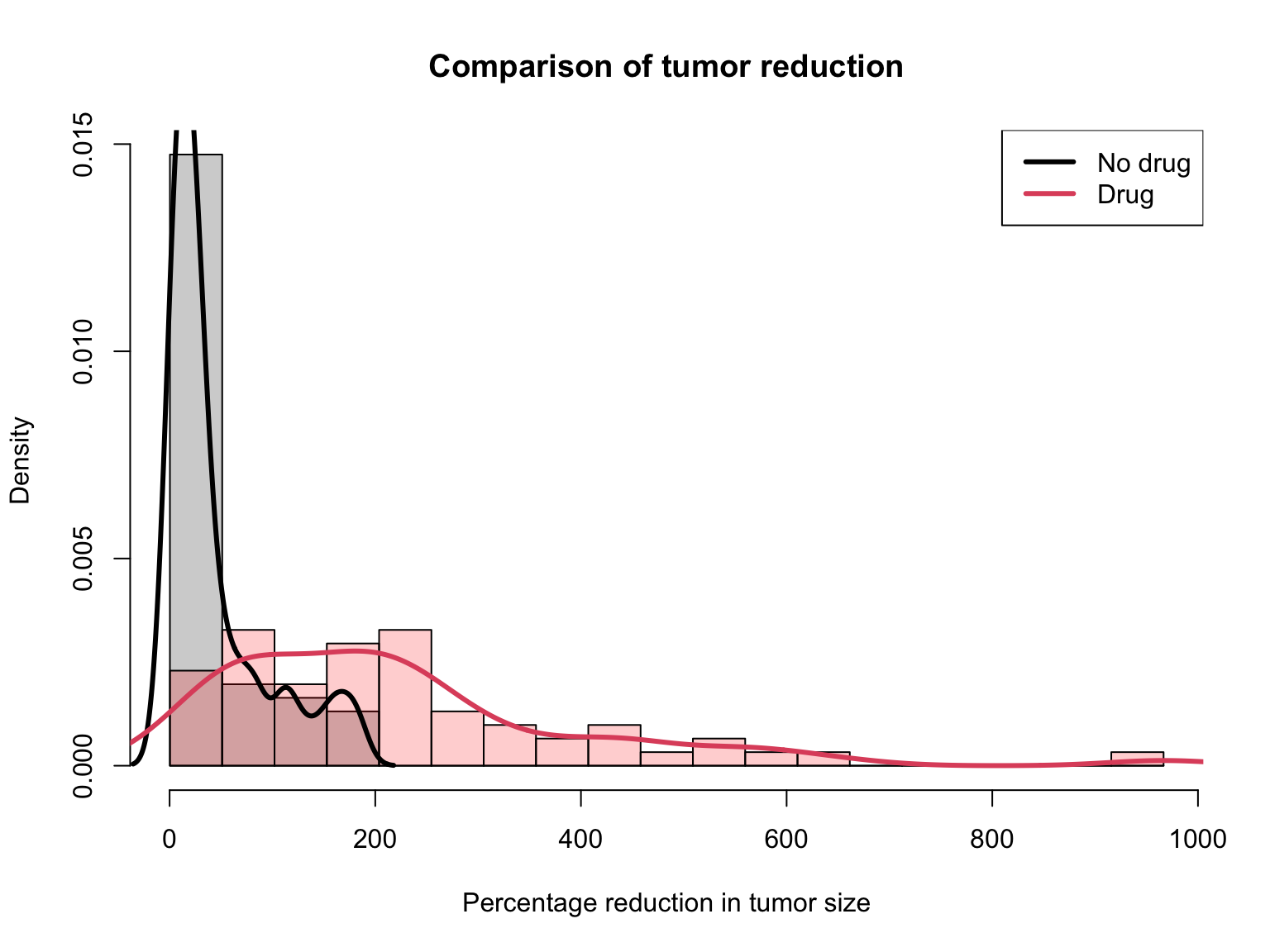# Simulation

Tuesday October 26, 2021

# Last week: Functions

• Function: formal encapsulation of a block of code; generally makes your code easier to understand, to work with, and to modify
• Functions are absolutely critical for writing (good) code for medium or large projects
• A function’s structure consists of three main parts: inputs, body, and output
• R allows the function designer to specify default values for any of the inputs
• R doesn’t allow the designer to return multiple outputs, but can return a list
• Side effects are things that happen as a result of a function call, but that aren’t returned as an output
• Top-down design means breaking a big task into small parts, implementing each of these parts, and then putting them together

# Part I

Simulation basics

# Why simulate?

R gives us unique access to great simulation tools (unique compared to other languages). Why simulate? Welcome to the 21st century! Two reasons:

• Often, simulations can be easier than hand calculations
• Often, simulations can be made more realistic than hand calculations

# Sampling from a given vector

To sample from a given vector, use sample()

sample(x=letters, size=10) # Without replacement, the default
##   "n" "j" "x" "u" "w" "f" "r" "p" "b" "h"
sample(x=c(0,1), size=10, replace=TRUE) # With replacement
##   1 1 1 1 1 0 0 1 1 0
sample(x=10) # Arguments set as x=1:10, size=10, replace=FALSE
##    4 10  1  2  9  8  5  3  6  7

# Random number generation

To sample from a normal distribution, we have the utility functions:

• rnorm(): generate normal random variables
• pnorm(): normal distribution function, $$\Phi(x)=P(Z \leq x)$$
• dnorm(): normal density function, $$\phi(x)=\Phi'(x)$$
• qnorm(): normal quantile function, $$q(y)=\Phi^{-1}(y)$$, i.e., $$\Phi(q(y))=y$$

Replace “norm” with the name of another distribution, all the same functions apply. E.g., “t”, “exp”, “gamma”, “chisq”, “binom”, “pois”, etc.

# Random number examples

Standard normal random variables (mean 0 and variance 1)

n = 100
z = rnorm(n, mean=0, sd=1) # These are the defaults for mean, sd
mean(z)  # Check: sample mean is approximately 0
##  0.07550971
var(z)   # Check: sample variance is approximately 1
##  1.0815

# Estimated distribution function

To compute empirical cumulative distribution function (ECDF)—the standard estimator of the cumulative distribution function (CDF)—use ecdf()

x = seq(-3, 3, length=100)
ecdf.fun = ecdf(z) # Create the ECDF
class(ecdf.fun) # It's a function!
##  "ecdf"     "stepfun"  "function"
ecdf.fun(0)
##  0.54
# We can plot it
plot(x, ecdf.fun(x), lwd=2, col="red", type="l", ylab="CDF", main="ECDF")
lines(x, pnorm(x), lwd=2)
legend("topleft", legend=c("Empirical", "Actual"), lwd=2,
col=c("red","black"))# Interlude: Kolmogorov-Smirnov test

One of the most celebrated tests in statistics is due to Kolmogorov in 1933. The Kolmogorov-Smirnoff (KS) statistic is: $\sqrt{\frac{n}{2}} \sup_{x} |F_n(x)-G_n(x)|$ Here $$F_n$$ is the ECDF of $$X_1,\ldots,X_n \sim F$$, and $$G_n$$ is the ECDF of $$Y_1,\ldots,Y_n \sim G$$. Under the null hypothesis $$F=G$$ (two distributions are the same), as $$n \to \infty$$, the KS statistic approaches the supremum of a Brownian bridge: $\sup_{t \in [0,1]} |B(t)|$

Here $$B$$ is a Gaussian process with $$B(0)=B(1)=0$$, mean $$\mathbb{E}(B(t))=0$$ for all $$t$$, and covariance function $$\mathrm{Cov}(B(s), B(t)) = s(1-t)$$

n = 500
t = 1:n/n
Sig = t %o% (1-t)
Sig = pmin(Sig, t(Sig))
eig = eigen(Sig)
Sig.half = eig$vec %*% diag(sqrt(eig$val)) %*% t(eig$vec) B = Sig.half %*% rnorm(n) plot(t, B, type="l")Two remarkable facts about the KS test: 1. It is distribution-free, meaning that the null distribution doesn’t depend on $$F,G$$! 2. We can actually compute the null distribution and use this test, e.g., via ks.test(): ks.test(rnorm(n), rt(n, df=1)) # Normal versus t1 ## ## Two-sample Kolmogorov-Smirnov test ## ## data: rnorm(n) and rt(n, df = 1) ## D = 0.142, p-value = 8.365e-05 ## alternative hypothesis: two-sided ks.test(rnorm(n), rt(n, df=10)) # Normal versus t10 ## ## Two-sample Kolmogorov-Smirnov test ## ## data: rnorm(n) and rt(n, df = 10) ## D = 0.06, p-value = 0.3291 ## alternative hypothesis: two-sided # Estimated density function To compute histogram—a basic estimator of the density based on binning—use hist() hist.obj = hist(z, breaks=30, plot=FALSE) class(hist.obj) # It's a list ##  "histogram" hist.obj$breaks # These are the break points that were used
##   -3.0 -2.8 -2.6 -2.4 -2.2 -2.0 -1.8 -1.6 -1.4 -1.2 -1.0 -0.8 -0.6 -0.4
##  -0.2  0.0  0.2  0.4  0.6  0.8  1.0  1.2  1.4  1.6  1.8  2.0  2.2  2.4
##   2.6  2.8
hist.obj\$density # These are the estimated probabilities
##   0.05 0.00 0.00 0.00 0.00 0.00 0.05 0.25 0.05 0.35 0.30 0.20 0.40 0.50
##  0.55 0.35 0.10 0.45 0.15 0.35 0.10 0.05 0.30 0.20 0.05 0.05 0.10 0.00
##  0.05
# We can plot it
plot(hist.obj, col="pink", freq=FALSE, main="Histogram")
lines(x, dnorm(x), lwd=2)
legend("topleft", legend=c("Histogram", "Actual"), lwd=2,
col=c("pink","black"))# Part II

Pseudorandomness and seeds

# Same function call, different results

Not surprisingly, we get different draws each time we call rnorm()

mean(rnorm(n))
##  0.01911518
mean(rnorm(n))
##  -0.07090431
mean(rnorm(n))
##  -0.01171319
mean(rnorm(n))
##  -0.03441602

# Is it really random?

Random numbers generated in R (in any language) are not “truly” random; they are what we call pseudorandom

• These are numbers generated by computer algorithms that very closely mimick “truly” random numbers
• The study of such algorithms is an interesting research area in its own right!
• The default algorithm in R (and in nearly all software languages) is called the “Mersenne Twister”
• Type ?Random in your R console to read more about this (and to read how to change the algorithm used for pseudorandom number generation, which you should never really have to do, by the way)

# Setting the random seed

All pseudorandom number generators depend on what is called a seed value

• This puts the random number generator in a well-defined “state”, so that the numbers it generates, from then on, will be reproducible
• The seed is just an integer, and can be set with set.seed()
• The reason we set it: so that when someone else runs our simulation code, they can see the same—albeit, still random—results that we do

# Seed examples

# Getting the same 5 random normals over and over
set.seed(0); rnorm(5)
##   1.2629543 -0.3262334  1.3297993  1.2724293  0.4146414
set.seed(0); rnorm(5)
##   1.2629543 -0.3262334  1.3297993  1.2724293  0.4146414
set.seed(0); rnorm(5)
##   1.2629543 -0.3262334  1.3297993  1.2724293  0.4146414
# Different seeds, different numbers
set.seed(1); rnorm(5)
##  -0.6264538  0.1836433 -0.8356286  1.5952808  0.3295078
set.seed(2); rnorm(5)
##  -0.89691455  0.18484918  1.58784533 -1.13037567 -0.08025176
set.seed(3); rnorm(5)
##  -0.9619334 -0.2925257  0.2587882 -1.1521319  0.1957828
# Each time the seed is set, the same sequence follows (indefinitely)
set.seed(0); rnorm(3); rnorm(2); rnorm(1)
##   1.2629543 -0.3262334  1.3297993
##  1.2724293 0.4146414
##  -1.53995
set.seed(0); rnorm(3); rnorm(2); rnorm(1)
##   1.2629543 -0.3262334  1.3297993
##  1.2724293 0.4146414
##  -1.53995
set.seed(0); rnorm(3); rnorm(2); rnorm(1)
##   1.2629543 -0.3262334  1.3297993
##  1.2724293 0.4146414
##  -1.53995

# Part III

Iteration and simulation

# Drug effect model

• Let’s start with a motivating example: suppose we had a model for the way a drug affected certain patients
• All patients will undergo chemotherapy. We believe those who aren’t given the drug experience a reduction in tumor size of percentage $X_{\mathrm{no\,drug}} \sim 100 \cdot \mathrm{Exp}(\mathrm{mean}=R), \;\;\; R \sim \mathrm{Unif}(0,1)$
• And those who were given the drug experience a reduction in tumor size of percentage $X_{\mathrm{drug}} \sim 100 \cdot \mathrm{Exp}(\mathrm{mean}=2)$ (Here $$\mathrm{Exp}$$ denotes the exponential distribution, and $$\mathrm{Unif}$$ the uniform distribution)

# What would you do?

What would you do if you had such a model, and your scientist collaborators asked you: how many patients would we need to have in each group (drug, no drug), in order to reliably see that the average reduction in tumor size is large?

• Answer used to be: get out your pen and paper, make some approximations
• Answer is now: simulate from the model, no approximations required!

# So, let’s simulate!

# Simulate, supposing 60 subjects in each group
set.seed(0)
n = 60
mu.drug = 2
mu.nodrug = runif(n, min=0, max=1)
x.drug = 100*rexp(n, rate=1/mu.drug)
x.nodrug = 100*rexp(n, rate=1/mu.nodrug)
# Find the range of all the measurements together, and define breaks
x.range = range(c(x.nodrug,x.drug))
breaks = seq(min(x.range),max(x.range),length=20)

# Produce hist of the non drug measurements, then drug measurements on top
hist(x.nodrug, breaks=breaks, probability=TRUE, xlim=x.range,
col="lightgray", xlab="Percentage reduction in tumor size",
main="Comparison of tumor reduction")

# Plot a histogram of the drug measurements, on top
hist(x.drug, breaks=breaks, probability=TRUE, col=rgb(1,0,0,0.2), add=TRUE)

# Draw estimated densities on top, for each dist
lines(density(x.nodrug), lwd=3, col=1)
lines(density(x.drug), lwd=3, col=2)
legend("topright", legend=c("No drug","Drug"), lty=1, lwd=3, col=1:2)# Repetition and reproducibility

• One single simulation is not always trustworthy (depends on the situation, of course)
• In general, simulations should be repeated and aggregate results reported—requires iteration!
• To make random number draws reproducible, we must set the seed with set.seed()
• More than this, to make simulation results reproducible, we need to follow good programming practices
• Gold standard: any time you show a simulation result (a figure, a table, etc.), you have code that can be run (by anyone) to produce exactly the same result

# Iteration and simulation (and functions): good friends

• Writing a function to complete a single run of your simulation is often very helpful
• This allows the simulation itself to be intricate (e.g., intricate steps, several simulation parameters), but makes running the simulation simple
• Then you can use iteration to run your simulation over and over again
• Good design practice: write another function for this last part (running your simulation many times)

# Code sketch

Consider the code below for a generic simulation. Think about how you would frame this for the drug effect example, which you’ll revisit in lab

# Function to do one simulation run
one.sim = function(param1, param2=value2, param3=value3) {
# Possibly intricate simulation code goes here
}

# Function to do repeated simulation runs
rep.sim = function(nreps, param1, param2=value2, param3=value3, seed=NULL) {
# Set the seed, if we need to
if(!is.null(seed)) set.seed(seed)

# Run the simulation over and over
sim.objs = vector(length=nreps, mode="list")
for (r in 1:nreps) {
sim.objs[r] = one.sim(param1, param2, param3)
}

# Aggregate the results somehow, and then return something
}

# Saving results

Sometimes simulations take a long time to run, and we want to save intermediate or final output, for quick reference later

There two different ways of saving things from R (there are more than two, but here are two useful ones):

• saveRDS(): allows us to save single R objects (like a vector, matrix, list, etc.), in (say) .rds format. E.g.,

saveRDS(my.mat, file="my.matrix.rds")
• save(): allows us to save any number of R objects in (say) .rdata format. E.g.,

save(mat.x, mat.y, list.z, file="my.objects.rdata")

Note: there is a big difference between how these two treat variable names

Corresponding to the two different ways of saving, we have two ways of loading things into R:

• readRDS(): allows us to load an object that has been saved by savedRDS(), and assign a new variable name. E.g.,

my.new.mat = readRDS("my.matrix.rds")
• load(): allows us to load all objects that have been saved through save(), according to their original variables names. E.g.,

load("my.objects.rdata")

# Summary

• Running simulations is an integral part of being a statistician in the 21st century
• R provides us with a utility functions for simulations from a wide variety of distributions
• To make your simulation results reproducible, you must set the seed, using set.seed()
• There is a natural connection between iteration, functions, and simulations
• Saving and loading results can be done in two formats: rds and rdata formats